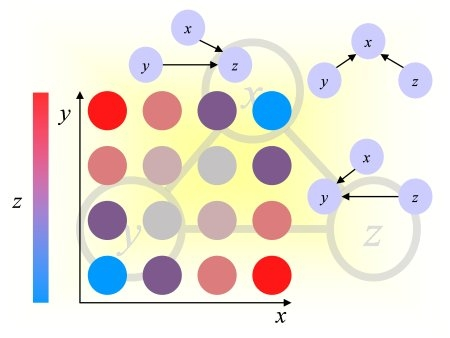Causality Workbench                                                             Challenges in Machine Learning[Back to list]

Contact: Denver Dash - Submitted: 2008-11-23 06:12 - Views : 7503 - [Edit entry]

### Abstract:

Summary:
This data represents a 9 variable (labeled X1...X9) dynamic system with several dynamic processes acting on qualitatively different time scales from one another. The goal is to learn a causal model of the system with the training data, and then correctly predict the effects of various manipulations on the system (using the testing data for a quantitative measure of performance). This dataset was meant to be both simple and extremely challenging. All relations are linear with independent Gaussian error terms. There are no hidden confounders. However, we believe the inter-related dynamic processes will make prediction of manipulations challenging.

Training Data:
The training data consists of 9 tab-separated text files (labelled X1.tsv, X2.tsv, etc.) one for each variable, and is arranged so that the rows in each file represent distinct time series for each variable (there are 10000 of these). That time series has been sampled at a few points in time after the exogenous variables of the system have been manipulated (all exogenous variables are held fixed for the duration of the time series). Specifically, the variables have been measured at the following discrete time intervals: t = [1,2,3,4,5,50,100,500,1000,2000,4000,10000], so there are 12 columns in each data file. Variables X8, X5 and X9 are all exogenous as can be verified by looking at X9.tsv, etc.

Test Data:
The test data is organized into several (6x9 = 54) data files labeled Xi-manipj.tsv (For example X2-manip3.tsv shows the values of variable X2 when X3 has been manipulated and held fixed). Each variable in the set of endogenous variables [X1,X2,X3,X4,X6,X7] is manipulated 100 times for the entire 10000 time-step duration of each time series while the remaining variables are measured once at each of the 12 predetermined time-intervals. Thus each Xi-manipj.tsv file has 100 rows and 12 columns, and there are 9 files for each variable manipulated from the set [X1,X2,X3,X4,X6,X7].

Evaluation:
The objective of this problem is to use the first set of data labeled X*.tsv to build a model which is then able to predict the effects of manipulation on the system as given by the X*-manipN.tsv files.

When predicting the effect of the manipulations, the goal is to predict the values of non-manipulated variables at times 5-10000 (columns 5 - 12) using the values of the previous times as input. For example, when predicting time 100 (column 7), you could use times 1,2,3,4,5,50 (columns 1-6) as input.

The output of the evaluation should be one table for each variable in the set [X1,X2,X3,X4,X6,X7] of manipulated variables. Each table should have 5 rows and 8 columns, one row for each variable in [X1,X2,X3,X4,X6,X7]\Xj, (where Xj is the manipulated variable), and one column for each time in the set [5,50,100,500,1000,2000,4000,10000]. The entry of the table is the RMS error (over the 100 runs) between the predicted value of the variable at that time and the actual value in the test data.